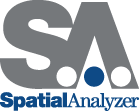SpatialAnalyzer

Measurement UncertaintyOften, measurement tasks require combining measurements from different instruments to assemble a total coordinate model of the object being measured. This requires either bundling or best-fitting the coordinate data into a common coordinate system. Once all the data is combined, the question is: What is the coordinate uncertainty for each point considering all the chaining necessary to build the model?

Currently, users just estimate the total uncertainty or assume it is only slightly larger than the uncertainty of each individual system. In addition, just determining the true coordinate uncertainty resulting from a single measurement system is not common. Often users rely on manufacturer specifications or rough estimates.

In addition, ISO compliance requires that all measurement are accompanied by a corresponding statement of measurement uncertainty. New River Kinematics has the tools to make this possible.

Our Solution

NRK has developed a series of tools to address measurement uncertainty. These tools give measurement system users a realistic measure of coordinate uncertainty regardless of the complexity of the setup of the amount of chaining or looping present in the system. There are three components to the uncertainty analysis tools:

1. 1. Characterizing the uncertainty of an individual measurement system.
2. 2. Applying this uncertainty to measurements to determine coordinate uncertainty for a single measurement system.
3. 3. Combining the uncertainties of individual measurement systems when they are chained together to produce a common coordinate system.

Individual Measurement System Uncertainty Characterization

SpatialAnalyzer® (SA) utilizes a bundle adjustment solution method to determine the uncertainty in a measurement system. To do this, simply measure a series of fixed (but unknown) targets from several instrument positions. Run this data through the SA bundle, and it will tell you the uncertainty in the instrument. This is based on the fact that even though the instrument has moved, the points should remain the same relative to one another. For a complete description of this method, see the publication, "A Practical Method for Evaluating Measurement System Uncertainty."

The result of this characterization is an uncertainty value for each of the instrument's output values. In the case of a theodolite, this is a horizontal and vertical angle uncertainty. For a laser tracker or a total station, there is also a distance uncertainty value. For a portable CMM arm, there are uncertainty values for each individual joint angle.

These uncertainty values for the instrument's measured values are then used to compute coordinate uncertainty.

Coordinate Uncertainty from Measurement System Uncertainty

Often, a user is most interested in the XYZ uncertainty of the coordinates they are measuring or are planning to measure. Since the bundle algorithm determines the uncertainty in terms of the instruments HVD measurements, it is necessary to map these values to coordinate uncertainty. By using the uncertainty results from the bundle in an error simulation algorithm, it is possible to calculate the coordinate uncertainty using the HVD instrument uncertainty.

To do this we simulate the uncertainty of the instrument’s values using a random distribution within the uncertainty bounds determined by the bundle. In this case, the horizontal uncertainty was computed at 0.96 arcseconds. This means that we apply a random distribution of errors to the horizontal measurement ranging from –0.96 to +0.96. We determine this distribution using both a Gaussian model as well as a rectangular model.

A simulation is performed using a large number of samples for each point. For each sample, random error is injected into all components of the measurements using the distributions discussed above. The result is a cloud of points surrounding the actual point. The following figure shows uncertainty clouds for a laser tracker and a total station:

Note that for three of the targets, BOTH instruments measured the points. The uncertainty clouds represent the error based on the computation of the point using BOTH instruments.

Combined Uncertainty from Chaining Individual Measurement Systems Together

It is common for a user to measure portions of of object with different instruments, then combine the measurements to produce a "WORLD" model. When this happens, the measurement sets form a linkage with a corresponding uncertainty. In the case shown below, laser trackers and total stations were used in a chain configuration to measure a large object.

In this case, the object being measured is a replacement steam generator for a nuclear power plant. The question is: What is the coordinate uncertainty of the points at the far end of the steam generator relative to the points at the near end? Obviously, it is not simple the uncertainty of any one instrument's measurements. Instead, it is a complex combination of the uncertainties in each instrument, and the tie-in point measurement geometry.

At NRK, we have developed software to quantify these uncertainty effects due to chaining effects. In addition, we are able to determine the uncertainty if you close the measurement loop. In this case that would mean using the last instrument in the chain to measure some common points with the first instrument. This reduces the uncertainty in the total measurement set.

These powerful tools allow you to determine the uncertainty you can expect for complex measurement jobs and optimize the measurement geometry and instrument placement to minimize measurement uncertainty.